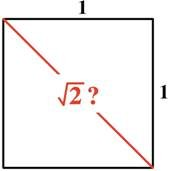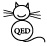# Irrational thoughts

by Burkard Polster and Marty Ross

The Age, 3 May 2010We have real sympathy for the writers of the draft national curriculum.

Okay, everybody please stop laughing and let us explain. Consider the following passage from the curriculum, an elaboration of Pythagoras' Theorem:

recognising that right-angled triangle calculations may generate results that can be integral, fractional or irrational numbers known as surds

True, this passage does not actively encourage sympathy. The expression "right-angled triangle calculations" is hopelessly vague, and what follows is either obvious or wrong.For the right-angled triangle pictured above, Pythagoras tells us that A2 + B2 = C2. There are whole number solutions to this equation: for example, A = 3, B = 4 and C = 5. It is also obvious that the solutions do not have to be whole numbers: for example, A = 3π B = 4π  and C = 5π .

Interestingly - and this is presumably the point of the quoted passage - the fact that A and B are whole numbers does not guarantee that C is as well. If A = 1 and B = 1 then it follows that C2 = 2, implying that C cannot be a whole number: C = 1 is too small and C = 2 is too big.

More mysteriously, if A = 1 and B = 1 then C also cannot be a fraction. Because of this we say that C is irrational, literally not a ratio. At this stage, it is common to declare the mystery solved by writing C = √2 or C = 1.414....

Not so fast.

First of all, though we may fail to find a fractional solution, how do we know that C cannot be a fraction? The question is not even raised in the curriculum. In practice, many textbooks instruct students to push the √-button on their calculator, and to then decide whether a number is irrational on the basis of the displayed digits. Such textbooks can be burned without loss.

Indeed, any emphasis upon identifying irrational numbers by peeking at their decimal digits is fundamentally misguided. A finite number of digits never suffices, and it is only for rare, contrived examples that one knows all the digits.

It seems never to be considered that one might prove that C is irrational. In fact there are beautiful proofs, completely accessible to school students. We have provided two such proofs here.

Returning to the curriculum quote, if A and B are whole numbers, can C ever be a fraction? Putting aside the fact that whole numbers can also be written as fractions, the answer is "No". The curriculum proposition, if it is not boringly obvious, goes awry by suggesting that true fractional solutions are indeed possible.

The above material can be tricky, though it is more a case of people tricking themselves. However, underlying it all is very tricky stuff, which has confused much cleverer people. This relates to the last part of the passage: "irrational numbers known as surds".

One question is, what does "surd" mean? Interestingly, "surd" probably doesn't mean anything at all, but that is a story for another day. Instead, let us concentrate upon "irrational".

We have concluded that the solution C to our original problem is not rational, but what is C? Physically or geometrically, we can think of C as the length of a hypotenuse, but to capture the numerical status of C is much more difficult. Writing C = √2 is no help, since √2 is exactly defined as the (positive) number which multiplied by itself results in 2; as an explanation, as a means to analyse C, this is pointlessly circular. And, C = 1.414... only helps if you can explain the dots. Good luck with that.

It is genuinely difficult to say anything sensible about irrational numbers. The Pythagoreans bumped into them about 2500 years ago, and for most of the time since these numbers have been regarded with suspicion, labelled as "artificial" and "inexplicable" and, well, "irrational". It is only about 150 years ago that irrational numbers were satisfactorily explained. It really is difficult, university-level stuff. And yet, irrational numbers are an unavoidable part of school mathematics.

So, really, we do have sympathy for the curriculum writers. They have made three or four brave attempts to say something sensible about this thorny topic. It is not entirely their fault that each time they have failed dismally.

#### Puzzle to Ponder

Feel free to suggest solutions in the comments section. Please also email us if you wish to be added to our email list.

Is π a surd? Is the triangle with side lengths 3 π , 4 π  and 5 π  covered by the curriculum quotation?

Burkard Polster teaches mathematics at Monash and is the university's resident mathemagician, mathematical juggler, origami expert, bubble-master, shoelace charmer, and Count von Count impersonator.

Marty Ross is a mathematical nomad. His hobby is smashing calculators with a hammer.

www.qedcat.com

Copyright 2004-∞All rights reserved.